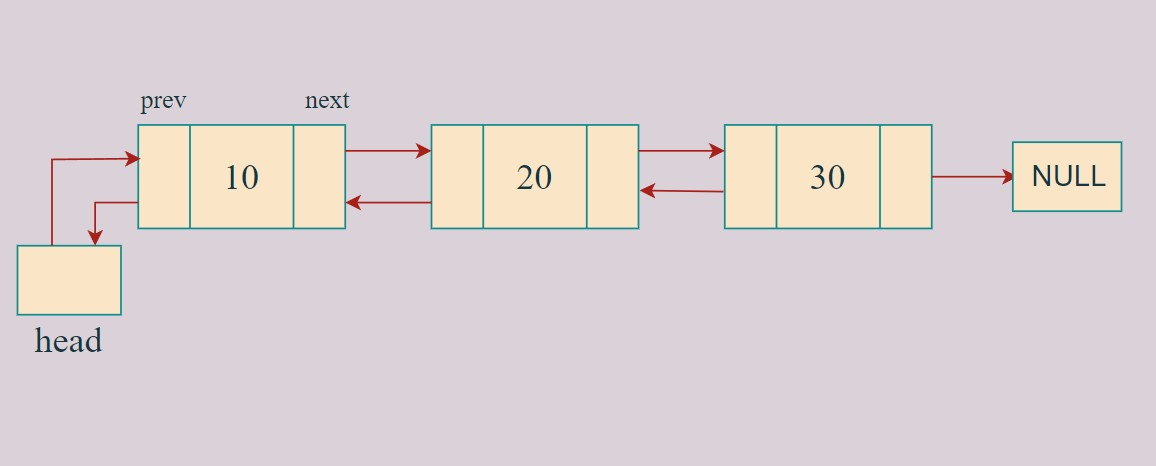# Doubly Linked List in C++

Doubly linked list: A doubly linked list is a little different from singly-linked lists.

It contains an extra pointer previous which points to the previous node of the linked lists.

Following are the important terms to understand the concept of a doubly-linked list :

1. node− Each node of a linked list can store a data called an element.
2. next − Each node of a linked list contains a pointer to the next node called  next.
3. prev− Each node of a linked list contains a pointer to the previous node called prev

## Doubly Linked List Representation:As per the above illustration, the following are the important points to be considered.

1. Doubly Linked List contains a pointer element called head.
2. Each node carries a data  and two pointer fields called next and prev.
3. Each node is linked with its next node using its next pointer.
4. Each node is linked with its previous node using its previous pointer.
5. The last node carries a pointer as null to mark the end of the list.

## Creating a node in doubly linked list in C++ :Code:

## C++ Code

``````#include<bits/stdc++.h>
using namespace std;

class node {
public:
int data;
node * next;        // pointer to the next node in dll
node * prev;	  // pointer to the previous node in dll

node(int val) 	  // constructor which declares the node.
{
data = val;
next = NULL;
prev = NULL;
}
};
``````

## Basic operations on doubly linked lists:

In a doubly-linked list, we perform the following operations:

1. Insertion
2. Deletion
3. Display

## Insertion:

In a double-linked list, the insertion operation can be performed in three ways as follows…

1. Inserting At Beginning of the list
2. Inserting At End of the list
3. Inserting At Specific location in the list

## Deletion :

In a double-linked list, the deletion operation can be performed in three ways as follows…

1. Deleting from Beginning of the list
2. Deleting from End of the list
3. Deleting a Specific Node

## Advantages of a Doubly Linked List

1. Allows us to iterate in both directions.
2. We can delete a node easily as we have access to its previous node.
3. Reversing is easy.
4. Can grow or shrink in size dynamically.

## Disadvantages of a Doubly Linked List

1. They use more memory than arrays because of the storage used by their pointers.

Complete Working Program with all operations:

## C++ Code

``````#include<bits/stdc++.h>

using namespace std;

class node {
public:
int data;
node * next;
node * prev;
node(int val) {
data = val;
next = NULL;
prev = NULL;
}
};

void insertAtTail(node * & head, int val) {
node * new_node = new node(val);
if (head == NULL) {
return;
}
node * temp = head;
while (temp -> next != NULL) {
temp = temp -> next;
}
temp -> next = new_node;
new_node -> prev = temp;
}

void insertAtHead(node * & head, int val) {
node * ptr = new node(val);
ptr -> next = head;
if (head != NULL) {
head -> prev = ptr;
}
}

void insertAtMiddle(node * & head, int val, int pos) {
node * ptr = new node(val);
node * temp = head;
for (int i = 0; i < pos - 2; i++) {
temp = temp -> next;
}
ptr -> next = temp -> next;
temp -> next = ptr;
ptr -> prev = temp;
if (ptr -> next != NULL) {
ptr -> next -> prev = ptr;
}
}

void deleteAtFirst(node * & head) {
node * todelete = head;
head -> prev = NULL;
delete todelete;
}

void deleteAtLast(node * & head) {
if (head -> next == NULL) {
return;
}
node * temp = head;
while (temp -> next != NULL) {
temp = temp -> next;
}
temp -> prev -> next = NULL;
free(temp);
}

void deleteAtposition(node * & head, int n) {
if (n == 1) {
return;
}
node * temp = head;
while (temp != NULL && n != 1) {
temp = temp -> next;
n--;
}
temp -> prev -> next = temp -> next;
if (temp -> next != NULL) {
temp -> next -> prev = temp -> prev;
}
}

void display(node * head) {
node * temp = head;
while (temp != NULL) {
cout << temp -> data << "-->";
temp = temp -> next;
}
cout << "NULL\n";
}

int main() {
node * head = NULL;
cout << "delete at the first:\n";
cout << "delete at the last:\n";
cout << "delete at the particular position:\n";

return 0;
}
``````

Output:

2–>3–>4–>5–>6–>NULL
1–>2–>3–>4–>5–>6–>NULL
1–>7–>2–>3–>4–>5–>6–>NULL
delete at the first:
7–>2–>3–>4–>5–>6–>NULL
delete at the last:
7–>2–>3–>4–>5–>NULL
delete at the particular position:
7–>2–>4–>5–>NULL

Special thanks to Ayush Pandey for contributing to this article on takeUforward. If you also wish to share your knowledge with the takeUforward fam, please check out this article